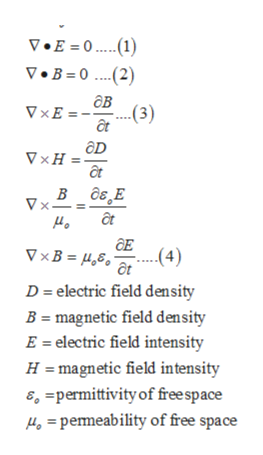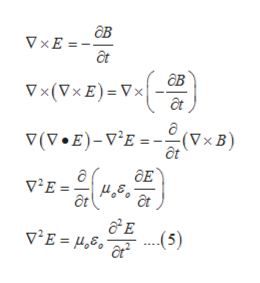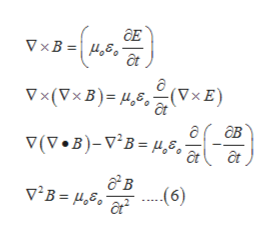# What will be the velocity of an electromagnetic wave in free space?

Question

What will be the velocity of an electromagnetic wave in free space?

check_circleExpert Solution
Step 1

Maxwell equation is used to calculate velocity of electromagnetic wave in free space,help_outlineImage TranscriptioncloseV.E = 0. 1) V.B=0(2) дв ..(3) VxE = VxH в д6,Е Vx- аE (4) VxB = ,8 D electric field density B magnetic field density E = electric field intensity H magnetic field intensity , =permittivity of free space 4=pemeability of free space fullscreen
Step 2

Equation of electric field is calculated as,help_outlineImage Transcriptioncloseав V xE = - дв Vx(Vx E) Vx a V(V.E)-VE=(VxB) ДЕ vE vE = 4,8. (5) fullscreen
Step 3

Equation of magnetic field...help_outlineImage TranscriptioncloseДE УxВ%3D н,6, Уx (VxB)- д,8. (YxE) дв (V-B)-v'в - д, 6, в (6) v'B= μδ , fullscreen

### Want to see the full answer?

See Solution

#### Want to see this answer and more?

Solutions are written by subject experts who are available 24/7. Questions are typically answered within 1 hour*

See Solution
*Response times may vary by subject and question
Tagged in

### Electrical Engineering